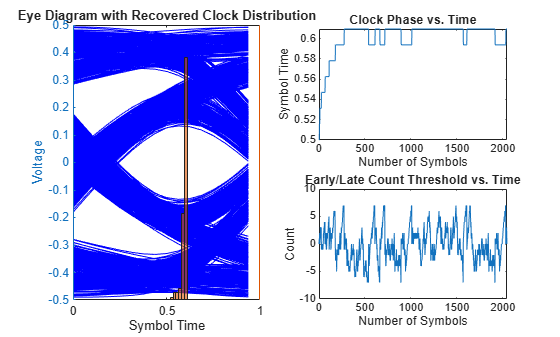# serdes.CDR

Performs clock data recovery function

## Description

The `serdes.CDR` System object™ provides clock sampling times and estimates data symbols at the receiver using a first order phase tracking CDR model. For more information, see Clock and Data Recovery in SerDes System.

To provide clock data locations:

1. Create the `serdes.CDR` object and set its properties.

2. Call the object with arguments, as if it were a function.

## Creation

### Syntax

``cdr = serdes.CDR``
``cdr = serdes.CDR(Name,Value)``

### Description

````cdr = serdes.CDR` returns a CDR object that determines the clock sampling times and estimates the data symbol according to the Bang-Bang CDR algorithm. It does not return or modify the incoming waveform.```
````cdr = serdes.CDR(Name,Value)` sets properties using one or more name-value pairs. Enclose each property name in quotes. Unspecified properties have default values.Example: `cdr = serdes.CDR('Count',8)` returns a CDR object with early or late CDR count threshold of 8.```

## Properties

expand all

Unless otherwise indicated, properties are nontunable, which means you cannot change their values after calling the object. Objects lock when you call them, and the `release` function unlocks them.

If a property is tunable, you can change its value at any time.

### Main

Early or late CDR count threshold to trigger a phase update, specified as a unitless real positive integer ≥4. Increasing the value of `Count` provides a more stable output clock phase at the expense of convergence speed. Because the bit decisions are made at the clock phase output, a more stable clock phase has a better bit error rate (BER).

`Count` also controls the bandwidth of the CDR which is approximately calculated by using the equation:

Data Types: `double`

Clock phase resolution, specified as a real scalar in fraction of symbol time. `Step` is the inverse of the number of phase adjustments in CDR.

Data Types: `double`

Clock phase offset, specified as a real scalar in the range [-0.5,0.5] in fraction of symbol time. `PhaseOffset` is used to manually shift clock probability distribution function (PDF) for better bit error rate (BER).

Data Types: `double`

Reference clock offset impairment, specified as a real scalar ≤300 in parts per million (ppm). `ReferenceOffset` is the deviation between transmitter oscillator frequency and receiver oscillator frequency.

Data Types: `double`

Sampling latch meta-stability voltage, specified as a real scalar in volts. If the data sample voltage lies within the region (+/-`Sensitivity`), there is a 50% probability of bit error..

Data Types: `double`

Time of a single symbol duration, specified as a real scalar in s.

Data Types: `double`

Uniform time step of the waveform, specified as a real scalar in s.

Data Types: `double`

Modulation scheme, specified as `2` or `4`.

Modulation ValueModulation Scheme
`2`Non-return to zero (NRZ)
`4`Four-level pulse amplitude modulation (PAM4)

Data Types: `double`

Input wave type form:

• `'Sample'` — A sample-by-sample input signal.

• `'Impulse'` — An impulse response input signal.

Data Types: `char`

## Usage

### Syntax

``y = cdr(x)``

### Description

``y = cdr(x)``

### Input Arguments

expand all

Input baseband signal. The input to the CDR must be applied as one sample at a time and not as a vector.

## Object Functions

To use an object function, specify the System object as the first input argument. For example, to release system resources of a System object named `obj`, use this syntax:

`release(obj)`

expand all

 `step` Run System object algorithm `release` Release resources and allow changes to System object property values and input characteristics `reset` Reset internal states of System object

## Examples

collapse all

This example shows how to recover clock distribution using `serdes.CDR` system object™.

Use a symbol time of `100` ps and `16` samples per symbol. The channel has `5` dB loss.

```SymbolTime = 100e-12; SamplesPerSymbol = 16; dt = SymbolTime/SamplesPerSymbol; loss = 5; chan = serdes.ChannelLoss('Loss',loss,'dt',dt,... 'TargetFrequency',1/SymbolTime/2,'RiseTime',SamplesPerSymbol/4*dt);```

Create a random data pattern using a pseudorandom binary sequence of order 10.

```ord = 10; %PRBS order nrz=prbs(ord,2^ord-1); nrzPattern = nrz(:)' - 0.5; %[0,1] --> [-0.5,0.5]; ChannelPulseResponse = impulse2pulse(chan.impulse, SamplesPerSymbol, dt); waveprbs = pulse2wave(ChannelPulseResponse(:,1),nrzPattern,SamplesPerSymbol); wave2 = [waveprbs; waveprbs];```

Create the CDR object that utilizes NRZ modulation scheme.

```CDR1 = serdes.CDR('Modulation',2,'Count',8,'Step',1/64,... 'SymbolTime',SymbolTime,'SampleInterval',dt);```

Initialize the outputs.

```phase = zeros(1,length(wave2)); CDRearlyLateCount = zeros(1,length(wave2));```

Feed the waveform one sample at a time through the CDR object.

```for ii = 1:length(wave2) [phase(ii), ~, optional] = CDR1(wave2(ii)); CDRearlyLateCount(ii) = optional.CDRearlyLateCount; end```

Plot the eye diagram with recovered clock distribution, clock phase vs. time, and early/late count threshold vs. time.

```t = (0:length(wave2)-1)/SamplesPerSymbol; teye = (0:SamplesPerSymbol-1)/SamplesPerSymbol; eyed = reshape(wave2,SamplesPerSymbol,[]); figure, subplot(2,2,[1,3]), yyaxis left, plot(teye,eyed, '-b'), title('Eye Diagram with Recovered Clock Distribution') xlabel('Symbol Time'), ylabel('Voltage') yyaxis right, histogram(phase,SamplesPerSymbol/2) set(gca,'YTick',[]) subplot(2,2,2), plot(t,phase) xlabel('Number of Symbols'), ylabel('Symbol Time'); title('Clock Phase vs. Time') subplot(224), plot(t,CDRearlyLateCount) xlabel('Number of Symbols'), ylabel('Count') title('Early/Late Count Threshold vs. Time')```## Extended Capabilities